# Spherically Symmetric Configurations Synopsis

Spherically Symmetric Configurations that undergo Adiabatic Compression/Expansion — adiabatic index,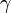$~\gamma$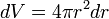$~dV = 4\pi r^2 dr$ and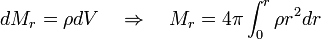$~dM_r = \rho dV ~~~\Rightarrow ~~~M_r = 4\pi \int_0^r \rho r^2 dr$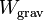$~W_\mathrm{grav}$$~=$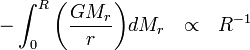$~- \int_0^R \biggl(\frac{GM_r}{r}\biggr) dM_r ~~ \propto ~~ R^{-1}$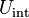$~U_\mathrm{int}$$~=$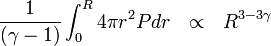$~\frac{1}{(\gamma -1)} \int_0^R 4\pi r^2 P dr ~~ \propto ~~ R^{3-3\gamma}$

Equilibrium Structure

Detailed Force Balance

Free-Energy Analysis

Given a barotropic equation of state,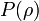$~P(\rho)$, solve the equation of

Hydrostatic Balance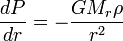$~\frac{dP}{dr} = - \frac{GM_r \rho}{r^2}$

for the radial density distribution,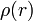$~\rho(r)$.

The Free-Energy is,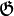$~\mathfrak{G}$$~=$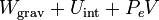$~W_\mathrm{grav} + U_\mathrm{int} + P_eV$$~=$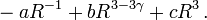$~-a R^{-1} + bR^{3-3\gamma}+ cR^3 \, .$

Therefore, also,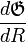$~\frac{d\mathfrak{G}}{dR}$$~=$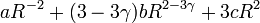$~aR^{-2} +(3-3\gamma)bR^{2-3\gamma} + 3cR^2$$~=$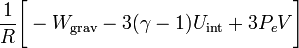$~\frac{1}{R}\biggl[ -W_\mathrm{grav} - 3(\gamma-1)U_\mathrm{int} + 3P_eV\biggr]$

Equilibrium configurations exist at extrema of the free-energy function, that is, they are identified by setting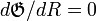$~d\mathfrak{G}/dR = 0$. Hence, equilibria are defined by the condition,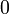$~0$$~=$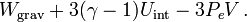$~W_\mathrm{grav} + 3(\gamma-1)U_\mathrm{int} - 3P_eV\, .$
Virial Equilibrium

Multiply the hydrostatic-balance equation through by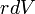$~rdV$ and integrate over the volume:$~0$$~=$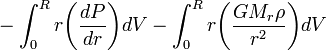$~-\int_0^R r\biggl(\frac{dP}{dr}\biggr)dV - \int_0^R r\biggl(\frac{GM_r \rho}{r^2}\biggr)dV$$~=$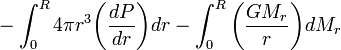$~-\int_0^R 4\pi r^3 \biggl(\frac{dP}{dr}\biggr) dr - \int_0^R \biggl(\frac{GM_r}{r}\biggr)dM_r$$~=$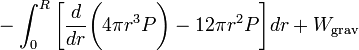$~-\int_0^R\biggl[ \frac{d}{dr}\biggl( 4\pi r^3P \biggr) - 12\pi r^2 P\biggr] dr + W_\mathrm{grav}$$~=$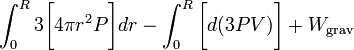$~\int_0^R 3\biggl[ 4\pi r^2 P \biggr]dr - \int_0^R \biggl[ d(3PV)\biggr] + W_\mathrm{grav}$$~=$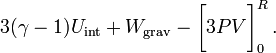$~3(\gamma-1)U_\mathrm{int} + W_\mathrm{grav} - \biggl[ 3PV \biggr]_0^R \, .$

Stability Analysis

Perturbation Theory

Free-Energy Analysis

Given the radial profile of the density and pressure in the equilibrium configuration, solve the eigenvalue problem defined by the,$~0$$~=$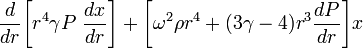$~ \frac{d}{dr}\biggl[ r^4 \gamma P ~\frac{dx}{dr} \biggr] +\biggl[ \omega^2 \rho r^4 + (3\gamma - 4) r^3 \frac{dP}{dr} \biggr] x$

to find one or more radially dependent, radial-displacement eigenvectors,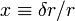$~x \equiv \delta r/r$, along with (the square of) the corresponding oscillation eigenfrequency,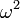$~\omega^2$.

The second derivative of the free-energy function is,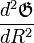$~\frac{d^2 \mathfrak{G}}{dR^2}$$~=$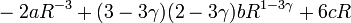$~ -2aR^{-3} + (3-3\gamma)(2-3\gamma)b R^{1-3\gamma} + 6cR$$~=$$~\frac{1}{R^2}\biggl[ 2W_\mathrm{grav} - 3(\gamma-1)(2-3\gamma)U_\mathrm{int} + 6P_e V \biggr] \, .$

Evaluating this second derivative for an equilibrium configuration — that is by calling upon the (virial) equilibrium condition to set the value of the internal energy — we have,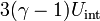$~3(\gamma-1)U_\mathrm{int}$$~=$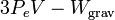$~3P_e V - W_\mathrm{grav}$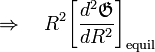$~\Rightarrow~~~ R^2 \biggl[\frac{d^2\mathfrak{G}}{dR^2}\biggr]_\mathrm{equil}$$~=$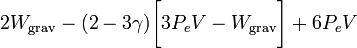$~2W_\mathrm{grav} - (2-3\gamma)\biggl[3P_e V - W_\mathrm{grav} \biggr] + 6P_e V$$~=$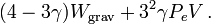$~(4-3\gamma)W_\mathrm{grav} + 3^2\gamma P_e V \, .$

Variational Principle

Multiply the LAWE through by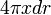$~4\pi x dr$, and integrate over the volume of the configuration gives the,

Governing Variational Relation$~0$$~=$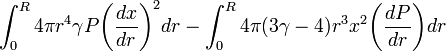$~ \int_0^R 4\pi r^4 \gamma P \biggl(\frac{dx}{dr}\biggr)^2 dr - \int_0^R 4\pi (3\gamma - 4) r^3 x^2 \biggl( \frac{dP}{dr} \biggr) dr$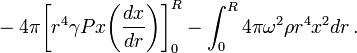$~ - 4\pi \biggr[r^4 \gamma Px \biggl(\frac{dx}{dr}\biggr) \biggr]_0^R - \int_0^R 4\pi \omega^2 \rho r^4 x^2 dr \, .$$~=$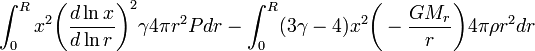$~ \int_0^R x^2 \biggl(\frac{d\ln x}{d\ln r}\biggr)^2 \gamma 4\pi r^2P dr - \int_0^R (3\gamma - 4)x^2 \biggl( - \frac{GM_r}{r} \biggr) 4\pi \rho r^2 dr$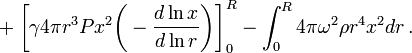$~ + \biggr[\gamma 4\pi r^3 Px^2 \biggl(-\frac{d\ln x}{d\ln r}\biggr) \biggr]_0^R - \int_0^R 4\pi \omega^2 \rho r^4 x^2 dr \, .$

Now, by setting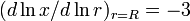$~(d\ln x/d\ln r)_{r=R} = -3$, we can ensure that the pressure fluctuation is zero and, hence,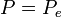$~P = P_e$ at the surface, in which case this relation becomes,$~\omega^2$$~=$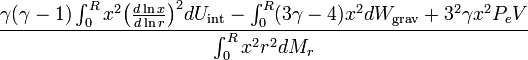$~ \frac{\gamma (\gamma -1) \int_0^R x^2 \bigl(\frac{d\ln x}{d\ln r}\bigr)^2 dU_\mathrm{int} - \int_0^R (3\gamma - 4)x^2 dW_\mathrm{grav} + 3^2 \gamma x^2 P_eV}{ \int_0^R x^2 r^2 dM_r}$

Approximation:   Homologous Expansion/Contraction

If we guess that radial oscillations about the equilibrium state involve purely homologous expansion/contraction, then the radial-displacement eigenfunction is,$~x$ = constant, and the governing variational relation gives,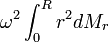$~\omega^2 \int_0^R r^2 dM_r$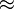$~\approx$$~ (4- 3\gamma) W_\mathrm{grav}+ 3^2 \gamma P_eV \, .$